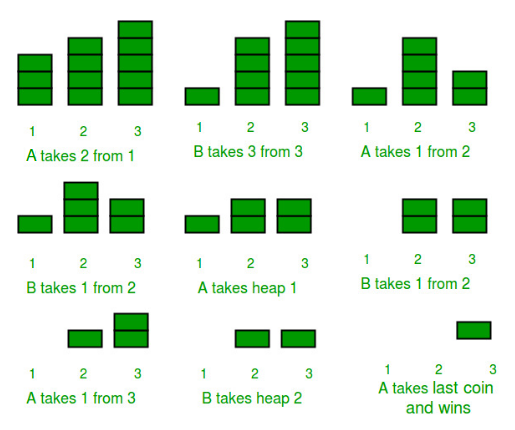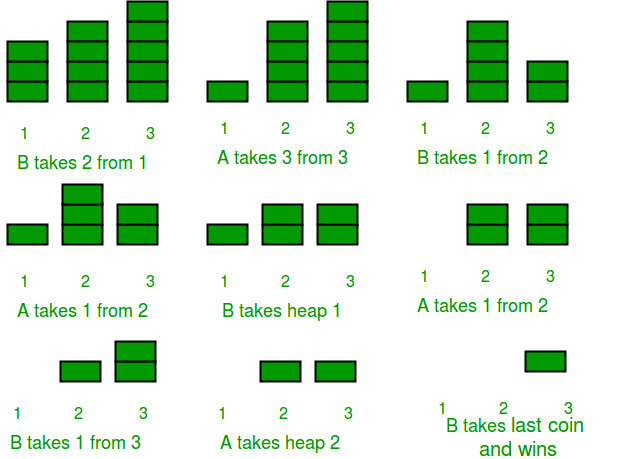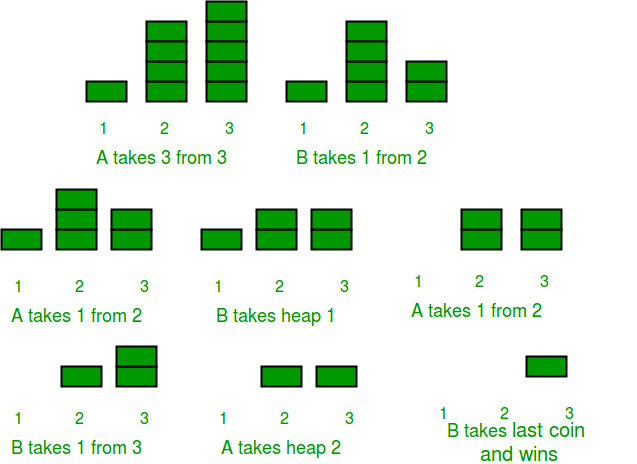Related Articles

# Combinatorial Game Theory | Set 2 (Game of Nim)

• Difficulty Level : Medium
• Last Updated : 04 May, 2020

We strongly recommend to refer below article as a prerequisite of this.

Combinatorial Game Theory | Set 1 (Introduction)

In this post, Game of Nim is discussed. The Game of Nim is described by the following rules-

Given a number of piles in which each pile contains some numbers of stones/coins. In each turn, a player can choose only one pile and remove any number of stones (at least one) from that pile. The player who cannot move is considered to lose the game (i.e., one who take the last stone is the winner).

For example, consider that there are two players- A and B, and initially there are three piles of coins initially having 3, 4, 5 coins in each of them as shown below. We assume that first move is made by A. See the below figure for clear understanding of the whole game play.A Won the match (Note: A made the first move)

So was A having a strong expertise in this game ? or he/she was having some edge over B by starting first ?

Let us now play again, with the same configuration of the piles as above but this time B starting first instead of A.B Won the match (Note: B made the first move)

By the above figure, it must be clear that the game depends on one important factor – Who starts the game first ?

So does the player who starts first will win everytime ?
Let us again play the game, starting from A , and this time with a different initial configuration of piles. The piles have 1, 4, 5 coins initially.

Will A win again as he has started first ? Let us see.A made the first move, but lost the Game.

So, the result is clear. A has lost. But how? We know that this game depends heavily on which player starts first. Thus, there must be another factor which dominates the result of this simple-yet-interesting game. That factor is the initial configuration of the heaps/piles. This time the initial configuration was different from the previous one.

So, we can conclude that this game depends on two factors-

1. The player who starts first.
2. The initial configuration of the piles/heaps.

In fact, we can predict the winner of the game before even playing the game !

Nim-Sum : The cumulative XOR value of the number of coins/stones in each piles/heaps at any point of the game is called Nim-Sum at that point.

“If both A and B play optimally (i.e- they don’t make any mistakes), then the player starting first is guaranteed to win if the Nim-Sum at the beginning of the game is non-zero. Otherwise, if the Nim-Sum evaluates to zero, then player A will lose definitely.”

For the proof of the above theorem, see- https://en.wikipedia.org/wiki/Nim#Proof_of_the_winning_formula

Optimal Strategy :

Couple of deductions about bitwise XOR necessary for understanding the Optimal Strategy:

• If the XOR sum of ‘n’ numbers is already zero then there is no possibility to make the XOR sum zero by single reduction of a number.
• If the XOR sum of ‘n’ numbers is non-zero then there is at least a single approach by which if you reduce a number, the XOR sum is zero.

Initially two cases could exist.

Case 1: Initial Nim Sum is zero
As we know, in this case if played optimally B wins, which means B would always prefer to have Nim sum of zero for A‘s turn.
So, as the Nim Sum is initially zero, whatever number of items A removes the new Nim Sum would be non-zero (as mentioned above). Also, as B would prefer Nim sum of zero for A‘s turn, he would then play a move so as to make the Nim Sum zero again (which is always possible, as mentioned above).
The game will run as long as there are items in any of the piles and in each of their respective turns A would make Nim sum non-zero and B would make it zero again and eventually there will be no elements left and B being the one to pick the last wins the game.

It is evident by above explanation that the optimal strategy for each player is to make the Nim Sum for his opponent zero in each of their turn, which will not be possible if it’s already zero.

Case 2: Initial Nim Sum is non-zero
Now going by the optimal approach A would make the Nim Sum to be zero now (which is possible as the initial Nim sum is non-zero, as mentioned above). Now, in B‘s turn as the nim sum is already zero whatever number B picks, the nim sum would be non-zero and A can pick a number to make the nim sum zero again. This will go as long as there are items available in any pile.
And A will be the one to pick the last item.

So, as discussed in the above cases, it should be obvious now that Optimal strategy for any player is to make the nim sum zero if it’s non-zero and if it is already zero then whatever moves the player makes now, it can be countered.

Let us apply the above theorem in the games played above. In the first game A started first and the Nim-Sum at the beginning of the game was, 3 XOR 4 XOR 5 = 2, which is a non-zero value, and hence A won. Whereas in the second game-play, when the initial configuration of the piles were 1, 4, and 5 and A started first, then A was destined to lose as the Nim-Sum at the beginning of the game was 1 XOR 4 XOR 5 = 0 .

Implementation:

In the program below, we play the Nim-Game between computer and human(user)
The below program uses two functions
knowWinnerBeforePlaying() : : Tells the result before playing.
playGame() : plays the full game and finally declare the winner. The function playGame() doesn’t takes input from the human(user), instead it uses a rand() function to randomly pick up a pile and randomly remove any number of stones from the picked pile.

The below program can be modified to take input from the user by removing the rand() function and inserting cin or scanf() functions.

## C++

 `/* A C++ program to implement Game of Nim. The program``assumes that both players are playing optimally */``#include ``#include ``using` `namespace` `std;`` ` `#define COMPUTER 1``#define HUMAN 2`` ` `/* A Structure to hold the two parameters of a move`` ` `A move has two parameters-``1) pile_index = The index of pile from which stone is``                    ``going to be removed``2) stones_removed = Number of stones removed from the``                        ``pile indexed = pile_index */``struct` `move``{``    ``int` `pile_index;``    ``int` `stones_removed;``};`` ` `/*``piles[] -> Array having the initial count of stones/coins``            ``in each piles before the game has started.``n     -> Number of piles`` ` `The piles[] are having 0-based indexing*/`` ` `// A C function to output the current game state.``void` `showPiles (``int` `piles[], ``int` `n)``{``    ``int` `i;``    ``cout <<``"Current Game Status -> "``;``    ``for` `(i=0; i 0)``                ``non_zero_indices [count++] = i;`` ` `        ``(*moves).pile_index = (``rand``() % (count));``        ``(*moves).stones_removed =``                ``1 + (``rand``() % (piles[(*moves).pile_index]));``        ``piles[(*moves).pile_index] =``        ``piles[(*moves).pile_index] - (*moves).stones_removed;`` ` `        ``if` `(piles[(*moves).pile_index] < 0)``            ``piles[(*moves).pile_index]=0;``    ``}``    ``return``;``}`` ` `// A C function to play the Game of Nim``void` `playGame(``int` `piles[], ``int` `n, ``int` `whoseTurn)``{``    ``cout <<``"\nGAME STARTS\n\n"``;``    ``struct` `move moves;`` ` `    ``while` `(gameOver (piles, n) == ``false``)``    ``{``        ``showPiles(piles, n);`` ` `        ``makeMove(piles, n, &moves);`` ` `        ``if` `(whoseTurn == COMPUTER)``        ``{``            ``cout <<``"COMPUTER removes"` `<< moves.stones_removed << ``"stones from pile at index "` `             ``<< moves.pile_index << endl;``            ``whoseTurn = HUMAN;``        ``}``        ``else``        ``{``            ``cout <<``"HUMAN removes"``<< moves.stones_removed << ``"stones from pile at index "` `            ``<< moves.pile_index << endl;``            ``whoseTurn = COMPUTER;``        ``}``    ``}`` ` `    ``showPiles(piles, n);``    ``declareWinner(whoseTurn);``    ``return``;``}`` ` `void` `knowWinnerBeforePlaying(``int` `piles[], ``int` `n,``                            ``int` `whoseTurn)``{``    ``cout <<``"Prediction before playing the game -> "``;`` ` `    ``if` `(calculateNimSum(piles, n) !=0)``    ``{``        ``if` `(whoseTurn == COMPUTER)``            ``cout <<``"COMPUTER will win\n"``;``        ``else``            ``cout <<``"HUMAN will win\n"``;``    ``}``    ``else``    ``{``        ``if` `(whoseTurn == COMPUTER)``            ``cout <<``"HUMAN will win\n"``;``        ``else``            ``cout <<``"COMPUTER will win\n"``;``    ``}`` ` `    ``return``;``}`` ` `// Driver program to test above functions``int` `main()``{``    ``// Test Case 1``    ``int` `piles[] = {3, 4, 5};``    ``int` `n = ``sizeof``(piles)/``sizeof``(piles);`` ` `    ``// We will predict the results before playing``    ``// The COMPUTER starts first``    ``knowWinnerBeforePlaying(piles, n, COMPUTER);`` ` `    ``// Let us play the game with COMPUTER starting first``    ``// and check whether our prediction was right or not``    ``playGame(piles, n, COMPUTER);`` ` `    ``/*``    ``Test Case 2``    ``int piles[] = {3, 4, 7};``    ``int n = sizeof(piles)/sizeof(piles);`` ` `    ``// We will predict the results before playing``    ``// The HUMAN(You) starts first``    ``knowWinnerBeforePlaying (piles, n, COMPUTER);`` ` `    ``// Let us play the game with COMPUTER starting first``    ``// and check whether our prediction was right or not``    ``playGame (piles, n, HUMAN); */`` ` `    ``return``(0);``}`` ` `// This code is contributed by shivanisinghss2110`

## C

 `/* A C program to implement Game of Nim. The program``   ``assumes that both players are playing optimally */``#include ``#include ``#include `` ` `#define COMPUTER 1``#define HUMAN 2`` ` `/* A Structure to hold the two parameters of a move`` ` ` ``A move has two parameters-``  ``1) pile_index = The index of pile from which stone is``                    ``going to be removed``  ``2) stones_removed = Number of stones removed from the``                        ``pile indexed = pile_index  */``struct` `move``{``    ``int` `pile_index;``    ``int` `stones_removed;``};`` ` `/*`` ``piles[] -> Array having the initial count of stones/coins``            ``in each piles before the game has started.`` ``n       -> Number of piles`` ` ` ``The piles[] are having 0-based indexing*/`` ` `// A C function to output the current game state.``void` `showPiles (``int` `piles[], ``int` `n)``{``    ``int` `i;``    ``printf` `(``"Current Game Status -> "``);``    ``for` `(i=0; i 0)``                ``non_zero_indices [count++] = i;`` ` `        ``(*moves).pile_index = (``rand``() % (count));``        ``(*moves).stones_removed =``                 ``1 + (``rand``() % (piles[(*moves).pile_index]));``        ``piles[(*moves).pile_index] =``         ``piles[(*moves).pile_index] - (*moves).stones_removed;`` ` `        ``if` `(piles[(*moves).pile_index] < 0)``            ``piles[(*moves).pile_index]=0;``    ``}``    ``return``;``}`` ` `// A C function to play the Game of Nim``void` `playGame(``int` `piles[], ``int` `n, ``int` `whoseTurn)``{``    ``printf``(``"\nGAME STARTS\n\n"``);``    ``struct` `move moves;`` ` `    ``while` `(gameOver (piles, n) == ``false``)``    ``{``        ``showPiles(piles, n);`` ` `        ``makeMove(piles, n, &moves);`` ` `        ``if` `(whoseTurn == COMPUTER)``        ``{``            ``printf``(``"COMPUTER removes %d stones from pile "``                   ``"at index %d\n"``, moves.stones_removed,``                   ``moves.pile_index);``            ``whoseTurn = HUMAN;``        ``}``        ``else``        ``{``            ``printf``(``"HUMAN removes %d stones from pile at "``                   ``"index %d\n"``, moves.stones_removed,``                   ``moves.pile_index);``            ``whoseTurn = COMPUTER;``        ``}``    ``}`` ` `    ``showPiles(piles, n);``    ``declareWinner(whoseTurn);``    ``return``;``}`` ` `void` `knowWinnerBeforePlaying(``int` `piles[], ``int` `n,``                             ``int` `whoseTurn)``{``    ``printf``(``"Prediction before playing the game -> "``);`` ` `    ``if` `(calculateNimSum(piles, n) !=0)``    ``{``        ``if` `(whoseTurn == COMPUTER)``            ``printf``(``"COMPUTER will win\n"``);``        ``else``            ``printf``(``"HUMAN will win\n"``);``    ``}``    ``else``    ``{``        ``if` `(whoseTurn == COMPUTER)``            ``printf``(``"HUMAN will win\n"``);``        ``else``            ``printf``(``"COMPUTER will win\n"``);``    ``}`` ` `    ``return``;``}`` ` `// Driver program to test above functions``int` `main()``{``    ``// Test Case 1``    ``int` `piles[] = {3, 4, 5};``    ``int` `n = ``sizeof``(piles)/``sizeof``(piles);`` ` `    ``// We will predict the results before playing``    ``// The COMPUTER starts first``    ``knowWinnerBeforePlaying(piles, n, COMPUTER);`` ` `    ``// Let us play the game with COMPUTER starting first``    ``// and check whether our prediction was right or not``    ``playGame(piles, n, COMPUTER);`` ` `    ``/*``    ``Test Case 2``    ``int piles[] = {3, 4, 7};``    ``int n = sizeof(piles)/sizeof(piles);`` ` `    ``// We will predict the results before playing``    ``// The HUMAN(You) starts first``    ``knowWinnerBeforePlaying (piles, n, COMPUTER);`` ` `    ``// Let us play the game with COMPUTER starting first``    ``// and check whether our prediction was right or not``    ``playGame (piles, n, HUMAN);    */`` ` `    ``return``(0);``}`

Output : May be different on different runs as random numbers are used to decide next move (for the loosing player).
```Prediction before playing the game -> COMPUTER will win

GAME STARTS

Current Game Status -> 3 4 5
COMPUTER removes 2 stones from pile at index 0
Current Game Status -> 1 4 5
HUMAN removes 3 stones from pile at index 1
Current Game Status -> 1 1 5
COMPUTER removes 5 stones from pile at index 2
Current Game Status -> 1 1 0
HUMAN removes 1 stones from pile at index 1
Current Game Status -> 1 0 0
COMPUTER removes 1 stones from pile at index 0
Current Game Status -> 0 0 0

COMPUTER won
```

References :
https://en.wikipedia.org/wiki/Nim

This article is contributed by Rachit Belwariar. If you like GeeksforGeeks and would like to contribute, you can also write an article and mail your article to contribute@geeksforgeeks.org. See your article appearing on the GeeksforGeeks main page and help other Geeks.